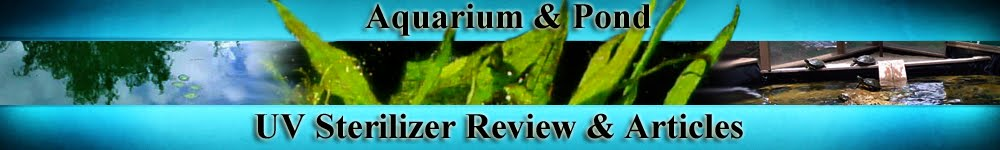. Aquarium and Pond UV Sterilizer, Clarifier Reviews; Problems

UV Sterilizer Reviews; Information Articles, Ideas, Comments, and Links to even more Resources about how UVC Sterilization works in Aquariums/ PondsAQUARIUM AND POND UV STERILIZER REVIEW/ ARTICLES; Information Articles (Posts), Ideas, Comments, & Links to even more Information about how UV (UVC) Sterilization works in Aquariums/ Ponds. For a COMPLETE up to date article about aquarium and pond uv sterilization, please visit this site:UV Sterilization in Aquariums and Ponds; How it works For all Articles, from basic to advanced, such as UVC, Watts, mW/cm2, please scroll down the Right Side Bar of this site for easy links

### UVC, Watts, Microwatts

Revised: 1/2/18

UVC, Watts, Microwatts, Joules, & Light Penetration

This article/post is intended to give some basic understanding between the relationship of watts, microwatts, joules of UVC energy and how this translates to UV Sterilizer effectiveness.

UVC Air Sterilization Devices

What is a Watt/Microwatt?

One Joule of energy = 1,000 milliWatt seconds = 1,000,000 microWatt seconds
One joule is the amount of energy required to perform the following actions:

• The work done by a force of one newton traveling through a distance of one meter (a newton is the unit of force equal to the amount of force required to accelerate a mass of one kilogram at a rate of one meter per second per second);
• The work required to move an electric charge of one coulomb* (the amount of electric charge transported by a current of 1 ampere in 1 second) through an electrical potential difference of one volt; or one coulomb volt, with the symbol C•V;
• The work done to produce power of one watt continuously for one second; or one watt second (compare kilowatt hour), with the symbol W•s. Thus a kilowatt hour is 3,600,000 joules or 3.6 megajoules;
• The kinetic energy of a 2 kg mass moving at a velocity of 1 m/s. The energy is linear in the mass but quadratic in the velocity, being given by E = ½mv²;
we measure UV-C intensity in Micro-Watts that strike one square centimeter of surface area.
Reference: What is a coulomb?

UVC Penetration;
A quote from an advanced sterilization article:
UV Sterilization; How a UV Sterilizer Works

"The emission or light intensity of a UVC germicidal light bulb is usually expressed in a term called "microwatts per square centimeter" (Mw/cm2). The maximum intensity provided by a single UV-C Bulb is at its surface.
So, if we calculate the surface area of the UVC lamp and only use that area which effectively emits UVC light rays, the effective area of UVC transmission will be established. Basic mathematics will show that the surface area of a cylindrical tube is ‘pie’ D L.

Next extrapolate this effective area of UVC transmission as having a screen with squares 1 centimeter in size. Each of these cm2 areas now, for measurement purposes, emits a UVC lamp intensity measured in microwatts, in other words; the term microwatts/cm2. UVC light intensity decreasingly varies as the distance from the UVC light increases.

Put more simply (a non scientific analogy); The amount of wattage will also increase penetration, as a higher watt UV-C bulb will generally have more Mw/cm2."

See this product link for high output Straight Tube UV Bulbs:
Premium HO Straight Tube UV Lamps

"In my own experiments I have used 15 watt and 25 watt UVC bulbs in exactly the same unit (both were 18”), if wattage were only considered there would be a 60% increase in effectiveness, however I only observed a about a 25% increase.
When I used a 30 Watt UVC bulb in a unit with over twice the exposure as the 15 Watt, the kill rate more than doubled. From my experience, if you increase wattage (and Mw/cm2) you need to also increase the volume of water to maximize the higher watt bulb.
Experiments can also be safely conducted with standard household light bulbs to correlate penetration. For this start with a 7 watt clear bulb (such as a Christmas bulb) and place varying thicknesses of paper/ cardboard in front of the bulb and measure when penetration stops. Continue this with higher and higher wattage bulbs."The Diagram to the left can give a rough comparison of distance as per UVC energy as expressed by MW/cm2 in Air transmission.
The dose applied by an UV-C lamp installation is a function of the lamp output, the intensity factor, and time. As an equation; Intensity x Exposure time= microwatt seconds/cm2.

As an example, a 9 watt UVC lamp at one inch from the lamp is found by this formula:
9 x 127 = 1143 mW/cm2.
Since many bacteria such as Vibrio require a UVC exposure of 6500 mW/cm2 or more, this means an exposure time of 5.68 seconds is required to kill this pathogen

Now let me point out that even though I have published this diagram, please use this as a rough guide only, as I have found inaccuracies in it. To be more blunt; I have found the distance, wattage, and flow rate to be the MOST IMPORTANT factors in determining exposure/effectiveness. This diagram is STATIC and does NOT take into consideration the dynamics of UVC radiation penetration for which I have yet to find a good formula to demonstrate this (even in University studies).

What is often missing in any equations I have seen is the dynamics of water flow geometry, actual water flow, and wattage. The bottom line is to use this table and others you might find elsewhere with “a grain of salt” noting that these are static and even then are flawed when true output via wattage is taken into consideration.